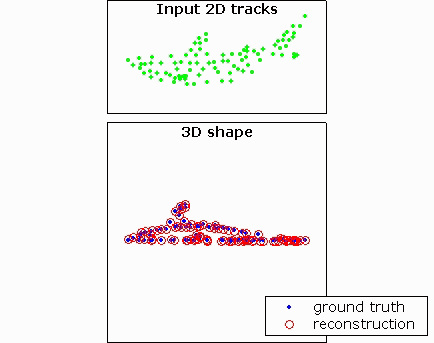# Learning Non-Rigid 3D Shape from 2D Motion

We present an algorithm for learning the time-varying shape of a non-rigid 3D object from uncalibrated 2D tracking data. We model shape motion as a rigid component (rotation and translation) combined with a non-rigid deformation. Reconstruction is ill-posed if arbitrary deformations are allowed. We constrain the problem by assuming that the object shape at each time instant is drawn from a Gaussian distribution. Based on this assumption, the algorithm simultaneously estimates 3D shape and motion for each time frame, learns the parameters of the Gaussian, and robustly fills-in missing data points. We then extend the algorithm to model temporal smoothness in object shape, thus allowing it to handle severe cases of missing data.paper: Learning Non-Rigid 3D Shape from 2D Motion, Lorenzo Torresani, Aaron Hertzmann and Christoph Bregler, NIPS 2003 (pdf)